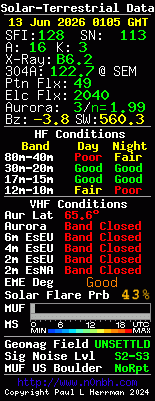G4URH - Calculations to Design Your Own AntennasIndex Balun Theory Beacon Listings Vhf Uhf Calculating Dbm Calculating Freq Coax Cable Spec Ic821h Mods Idiot Kenwood Ts2000 Kenwood Ts870 Kenwood Ts890 M0ebj S Match Prefix List Q Codes Radio Mods Uk Repeater Watt Converter# G4URH - Calculations to Design Your Own Antennas

Ground Planes

Halfwave Verticals

5/8th Wave Vertical

Dipoles and Inverted Vees

Ground Planes

The standard ground plane antenna consists of a vertical radiator which is insulated and centered between 4 horizontal surrounding radials which extend out from the base of the antenna. Each radial is evenly spaced 90 degrees or so from each other around the vertical element.

Enter the formula for the antenna calculation (normally 234/f):

divided by Freq. MHZ

The vertical length is feet.

The 4 radials are each feet.

Halfwave Verticals

The halfwave vertical antenna consists of a vertical radiator which is fed from the bottom of the antenna. A matching device should be added between the antenna and the feedline if you wish to use coax.

Enter the formula for the antenna calculation (normally 468/f):

Switch to:

divided by Freq. MHZ

Your halfwave antenna's vertical length is

The cubical quad antenna is a four sided antenna, with each side a quarter wave length long. Several factors influence the performance of the antenna, such as :

• The antenna height above the ground
• The diameter of the wire used
• Nearby structures to where the antenna is mounted
• Effects of other antennas or metal objects in the area
• The conductivity of the soil

Enter the formula for the antenna (normally 1005/f):

divided by Freq. MHZ

Each of the 4 sides are feet.

5/8th Wave Vertical Antenna

The 5/8 wave antenna consists of a vertical radiator fed at the bottom of the antenna. A matching device should be used if you feed it with coax.

Enter the formula for the antenna (normally 585/f or 178.308/f for metric):

Switch to:
divided by Freq. MHZ

Your 5/8 wave antenna's Vertical element is:

Dipole and Inverted Vee Antennas

Most familiar are the centerfed, halfwave dipoles and inverted vees that are so popular.

A basic formula for these types of antennas is below. For center fed, wire dipole antennas, the formula is 468/freq(mhz).

Enter the formula for the antenna:

divided by Freq. MHZ

Percent smaller for an Inverted Vee.

Your dipole's total length is feet. Each leg of the dipole is feet.

Your Inverted Vee's total length is feet. Each leg of the Inverted Vee is feet.